Eigenvalues & Eigenvectors - 1

# Eigenvalues & Eigenvectors - 1 - Notes | Study Mathematics for IIT JAM, CSIR NET, UGC NET - Mathematics

 1 Crore+ students have signed up on EduRev. Have you?

Introduction to Eigenvalues

Linear equations Ax = b come from steady state problems. Eigenvalues have their greatest importance in dynamic problems. The solution of du/dt = Au is changing with time- growing or decaying or oscillating. We can’t ﬁnd it by elimination. This chapter enters a new part of linear algebra, based on Ax = λx. All matrices in this chapter are square.

A good model comes from the powers A, A2, A3 ,... of a matrix. Suppose you need the hundredth power A100 . The starting matrix A becomes unrecognizable after a few steps, and A100 is very close to [.6 .6; .4 .4] :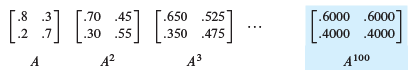A100 was found by using the eigenvalues of A, not by multiplying 100 matrices. Those eigenvalues (here they are 1 and 1=2) are a new way to see into the heart of a matrix.

To explain eigenvalues, we ﬁrst explain eigenvectors. Almost all vectors change direction, when they are multiplied by A. Certain exceptional vectors x are in the same direction as Ax. Those are the “eigenvectors”. Multiply an eigenvector by A, and the vector Ax is a number λ times the original x.

The basic equation is Ax = λx. The number λ is an eigenvalue of A.

The eigenvalue λ tells whether the special vector x is stretched or shrunk or reversed or left unchanged - when it is multiplied by A. We may ﬁnd λ = 2 or1/2  or -1 or 1. The eigen - value λ could be zero! Then Ax = 0x means that this eigenvector x is in the nullspace.

If is the identity matrix, every vector has Ax = x. All vectors are eigenvectors of I .
All eigenvalues “lambda” are λ = 1. This is unusual to say the least. Most 2 by 2 matrices have two eigenvector directions and two eigenvalues. We will show that det(A - λI) = 0.

This section will explain how to compute the x’s and λ’s. It can come early in the course because we only need the determinant of a 2 by 2 matrix. Let me use det(A - λI) = 0 to find the eigenvalues for this first example, and then derive it properly in equation (3).

Example 1 The matrix A has two eigenvalues λ = 1 and λ = 1/2. Look at det.(A - λI)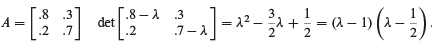I factored the quadratic into λ - 1 times λ - 1/2 , to see the two eigenvalues λ = 1 and λ = 1/2 . For those numbers, the matrix A - λI becomes singular (zero determinant). The eigenvectors x1 and x2 are in the nullspaces of A - I and A - 1/2 I.

(A - I)/x1 = 0 is Ax1 = x1 and the ﬁrst eigenvector is (.6,.4)

(A - 1/2 I)x2 = 0 is Ax= 1/2 x2 and the second eigenvector is (1, -1)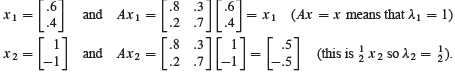If x1 is multiplied again by A, we still get x1 . Every power of A will give An x1 = x1 .
Multiplying x2 by A gave 1/2 x2 , and if we multiply again we get (1/2)2 times x2.

When A is squared, the eigenvectors stay the same. The eigenvalues are squared.

This pattern keeps going, because the eigenvectors stay in their own directions (Figure 6.1) and never get mixed. The eigenvectors of A100 are the same x1 and x2. The eigenvalues of A100 are 1100 = 1 and (1/2) 100 = very small number.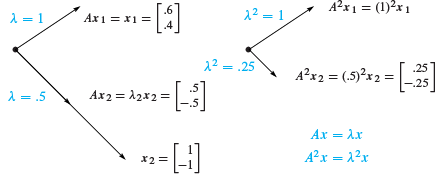Figure 6.1: The eigenvectors keep their directions. A2 has eigenvalues 12 and (.5)2 .
Other vectors do change direction. But all other vectors are combinations of the two eigenvectors. The ﬁrst column of A is the combination x1 + (.2)x2

Separate into eigenvectors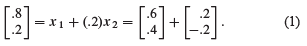Multiplying by A gives (.7,.3), the ﬁrst column of A2 . Do it separately for x1 and (.2)x2 . Of course Ax1 = x1 . And A multiplies x2 by its eigenvalue 1/2 :

Multiply each xi by λi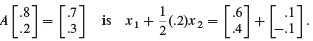Each eigenvector is multiplied by its eigenvalue, when we multiply by A. We didn’t need these eigenvectors to ﬁnd A2 . But it is the good way to do 99 multiplications. At every step x1 is unchanged and x2 is multiplied by (1/2) , so we have .(12) 99 :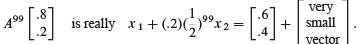This is the ﬁrst column of A100 . The number we originally wrote as :6000 was not exact. We left out (.2)(1/2)99 which wouldn’t show up for 30 decimal places.

The eigenvector x1 is a steady state that doesn't change (because λ1 = 1). The eigenvector x2 is a “decaying mode” that virtually disappears (because λ2 = .5). The higher the power of A, the closer its columns approach the steady state.

We mention that this particular A is a Markov matrix. Its entries are positive and every column adds to 1. Those facts guarantee that the largest eigenvalue is λ = 1 (as we found). Its eigenvector x1 = (.6, .4) is the steady state-which all columns of Ak will approach. Section 8.3 shows how Markov matrices appear in applications like Google.
For projections we can spot the steady state (λ = 1) and the nullspace (λ = 0).

Example 2 The projection matrix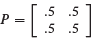has eigenvalues λ = 1 and λ = 0.

Its eigenvectors are x1 = (1, 1) and x2 = (1, -1). For those vectors, Px1 = x1 (steady state) and Px2 = 0 (nullspace). This example illustrates Markov matrices and singular matrices and (most important) symmetric matrices. All have special λ’s and x’s:

1. Each column ofadds to 1,so λ = 1 is an eigenvalue.

2. P is singular,so λ = 0 is an eigenvalue.

3. P is symmetric, so its eigenvectors (1,1) and (1, -1) are perpendicular.

The only eigenvalues of a projection matrix are 0 and 1. The eigenvectors for λ = 0 (which means Px = 0x) ﬁll up the nullspace. The eigenvectors for λ = 1 (which means Px = x) ﬁll up the column space. The nullspace is projected to zero. The column space projects onto itself. The projection keeps the column space and destroys the nullspace:

Project each part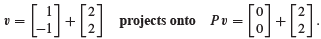Special properties of a matrix lead to special eigenvalues and eigenvectors. That is a major theme of this chapter (it is captured in a table at the very end).

Projections have λ = 0 and 1. Permutations have all |λ|= 1. The next matrix R (a reﬂection and at the same time a permutation) is also special.

Example 3  The reﬂection matrix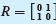has eigenvalues 1 and -1.

The eigenvector (1,1) is unchanged by R. The second eigenvector is (1, -1)—its signs are reversed by R. A matrix with no negative entries can still have a negative eigenvalue!
The eigenvectors for R are the same as for P , because reﬂection = 2(projection) - I :

R =  2P- I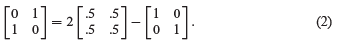Here is the point. If Px = λx then 2Px = 2λx. The eigenvalues are doubled when the matrix is doubled. Now subtract I x = x. The result is (2P - I)x = (2λ -1)x.
When a matrix is shifted by I , each λ is shifted by 1. No change in eigenvectors.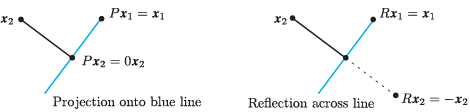Figure 6.2: Projections P have eigenvalues 1 and 0. Reflections R have λ = 1 and -1. A typical x changes direction, but not the eigenvectors x1 and x2.

Key idea: The eigenvalues of R and P are related exactly as the matrices are related:

The eigenvalues of R = 2P - I are 2(1) - 1 = 1 and 2(0) - 1 = -1.

The eigenvalues of R2 are λ2 . In this case R2 = I . Check (1)2 = 1 and (-1)2 = 1.

The Equation for the Eigenvalues

For projections and reflections we found λ’s and x’s by geometry: Px = x, Px = 0, Rx = -x. Now we use determinants and linear algebra. This is the key calculation in the chapter-almost every application starts by solving Ax = Ax.
First move λx to the left side. Write the equation Ax = λx as (A - λI)x = 0. The matrix A - λI times the eigenvector x is the zero vector. The eigenvectors make up the nullspace of A - λI. When we know an eigenvalue A, we find an eigenvector by solving (A - λI)x = 0.

Eigenvalues first. If (A - λI)x = 0 has a nonzero solution, A - λI is not invertible. The determinant of A - λI must be zero. This is how to recognize an eigenvalue λ:

Eigenvalues The number λ is an eigenvalue of A if and only if A - λI is singular:

det.(A - λI ) = 0:                     (3)

This “characteristic equation” det.(A - λI) = 0 involves only λ, not x. When A is n by n, the equation has degree n. Then A has n eigenvalues and each λ leads to x:

For each λ solve (A - λI)x = 0 or Ax = λx to ﬁnd an eigenvector x:

The document Eigenvalues & Eigenvectors - 1 - Notes | Study Mathematics for IIT JAM, CSIR NET, UGC NET - Mathematics is a part of the Mathematics Course Mathematics for IIT JAM, CSIR NET, UGC NET.
All you need of Mathematics at this link: Mathematics

## Mathematics for IIT JAM, CSIR NET, UGC NET

559 videos|198 docs
 Use Code STAYHOME200 and get INR 200 additional OFF

## Mathematics for IIT JAM, CSIR NET, UGC NET

559 videos|198 docs

Track your progress, build streaks, highlight & save important lessons and more!

,

,

,

,

,

,

,

,

,

,

,

,

,

,

,

,

,

,

,

,

,

,

,

,

,

,

,

;